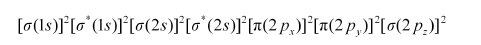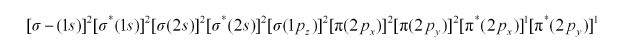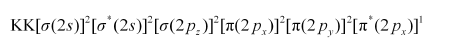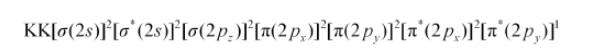# What is meant by the term bond order? Calculate the bond order of

Question:

What is meant by the term bond order? Calculate the bond order of: $\mathrm{N}_{2}, \mathrm{O}_{2}, \mathrm{O}_{2}^{+}$and $\mathrm{O}_{2}^{-}$.

Solution:

Bond order is defined as one half of the difference between the number of electrons present in the bonding and anti-bonding orbitals of a molecule.

If Na is equal to the number of electrons in an anti-bonding orbital, then Nb is equal to the number of electrons in a bonding orbital.

Bond order $=\frac{1}{2}\left(N_{\mathrm{b}}-N_{\mathrm{a}}\right)$

If Nb > Na, then the molecule is said be stable. However, if Nb ≤ Na, then the molecule is considered to be unstable.

Bond order of N2 can be calculated from its electronic configuration as:Number of bonding electrons, Nb = 10

Number of anti-bonding electrons, Na = 4

Bond order of nitrogen molecule $=\frac{1}{2}(10-4)$

= 3

There are 16 electrons in a dioxygen molecule, 8 from each oxygen atom. The electronic configuration of oxygen molecule can be written as:Since the 1s orbital of each oxygen atom is not involved in boding, the number of bonding electrons = 8 = Nand the number of anti-bonding electrons = 4 = Na.

Bond order $=\frac{1}{2}\left(N_{b}-N_{a}\right)$

$=\frac{1}{2}(8-4)$

= 2

Hence, the bond order of oxygen molecule is 2.

Similarly, the electronic configuration of $\mathrm{O}_{2}^{+}$can be written as:Nb = 8

Na = 3

Bond order of $\mathrm{O}_{2}^{+}=\frac{1}{2}(8-3)$

= 2.5

Thus, the bond order of $\mathrm{O}_{2}^{+}$is $2.5$.

The electronic configuration of $\mathrm{O}_{2}^{-}$ion will be:Bond order of $\mathrm{O}_{2}^{-}=\frac{1}{2}(8-5)$

= 1.5

Thus, the bond order of $\mathrm{O}_{2}^{-}$ion is $1.5$.